# Time to event data

## Time to event data

In health care research we often measure the time which elapses until some event occurs. This might be the time from diagnosis or start of treatment to death of cancer patients, the time to metastasis or to local recurrence of a tumour, the time to readmission to hospital after discharge of an asthma patient (Mitchell et al., 1994), the age at which breast-feeding ceased (Clements et al., 1997), the time from infertility treatment to conception (Luthra et al., 1982), the time to healing of a wound (Nelson et al., 2004), time to recurrence of a gallstone (Petroni et al., 2000), etc. We call such data time to event data. Sometimes the event is adverse, such as death, sometimes it is beneficial, such as healing.

Because of the examples of time to event data which were first studied, such data are often know as survival or failure time data. The terminal event, death, healing, etc., is called the endpoint. The statistical techniques developed to deal with them are known collectively as survival analysis.

The analysis of time to event data would not require any special methods if we knew the time to event of every subject. What makes time to event data difficult to analyse is that often we do not know the exact survival times of all cases. Some subjects will still be surviving when we want to analyse the data. For some events, such as conception or readmission to hospital, the event may never happen for some subjects. Furthermore, when cases have entered the study at different times, some of the recent entrants may be surviving, but have been observed for a short time only. Their observed survival time may be less than those subjects admitted early in the study and who have since experienced the event. When we know for some subjects only that the time to the event is greater than some value, we say that the data are censored. This also known as being withdrawn from follow-up.

Figure 1 shows the recruitment, time to event, and time to censoring of 10 subjects recruited into a hypothetical study.

Figure 1. Recruitment, time to event, and time to censoring of 10 subjects recruited into a study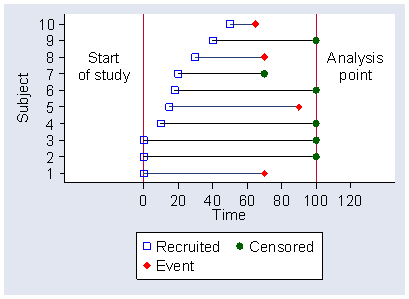d

Subjects 1, 2, and 3 are recruited at the beginning of the study. Subject 1 experiences the event, but subjects 2 and 3 do not. When we want to analyse the data, they have yet to experience the event. Subjects 4 and 6 are recruited after the start of the study and do not experience the event before the analysis point. Subject 5 is recruited after the start and does experience the event.

Subject 7 is recruited after the start of the study and is censored before the analysis point. There are many reasons why this might be. In a wound healing trial, the subject many be transferred to another treatment for clinical reasons. In a conception study, the subject might decide not to make any further attempt to conceive. In the study of Luthra et al. (1982), who compared the incidence of pregnancy in attenders at a sub-fertility clinic before and after laparoscopy and hydrotubation, one of the couples in the sample decided to divorce and cease trying for a family, another decided to adopt. Subject 7 is observed for a shorter time than subjects 1 and 5 take to experience the event. We cannot therefore simply say that we will look at whether subjects have experienced the event within the shortest observation time for a subject who has not experienced it, because we will lose most of our data.

## Kaplan Meier survival estimates

Some censored times may be shorter than some times to events. We overcome this difficulty by the construction of what we call a life table. A life table was originally a table showing what would happen to a cohort of hypothetical individuals from birth to death if the current death rates operated throughout their lives. It showed how many would still be alive at each age. Now we use the term to describe following a hypothetical cohort from any time point onwards. I shall show how this works through an example.

I shall use the time to healing of venous ulcers for patients recruited to the VenUS I trial (Nelson et al., 2004). VenUS I was a randomised trial of two types of bandage for treating venous leg ulcers: the test treatment, a four layer bandage (4LB), which gave elastic compression, and the control treatment, a short-stretch bandage (SSB), which gave inelastic compression. The endpoint is complete healing of the index ulcer and we observe the time to healing in days. Table 1 shows the times for the SSB group.

 7 H 28 H 45 H 59 H 86 H 119 H 189 H 244 H 413 H 671 H 8 C 28 H 45 H 60 H 90 C 124 H 189 H 273 C 417 C 672 C 10 H 28 H 47 H 62 H 90 C 125 H 191 H 284 H 428 C 691 C 12 H 28 H 48 C 63 H 90 H 126 H 195 H 286 H 461 H 742 C 13 H 30 C 48 H 63 H 91 H 127 H 195 H 309 C 465 H 746 C 14 H 30 H 49 H 63 H 92 H 134 H 199 H 322 H 483 H 790 C 15 H 31 C 49 H 63 H 94 H 135 H 201 H 332 H 493 C 791 C 20 H 34 H 50 H 63 H 97 H 142 C 202 C 334 C 504 C 858 C 20 H 35 H 50 H 63 H 99 H 146 H 210 H 336 H 517 H 869 C 21 H 35 H 50 H 68 C 101 H 147 H 212 H 343 H 525 H 886 C 21 H 36 H 50 H 68 H 104 H 148 H 212 H 364 H 549 H 924 C 21 H 36 H 53 C 70 H 106 H 151 H 214 H 369 C 579 H 955 C 21 H 41 H 53 H 70 H 112 H 154 C 216 H 369 C 585 C 22 H 41 H 56 H 73 C 112 H 154 H 218 H 370 C 602 H 24 H 41 H 56 H 73 H 113 H 158 H 224 H 377 C 612 C 24 H 42 H 56 H 73 H 114 H 174 H 232 H 378 C 648 H 25 H 42 H 57 C 77 H 115 H 179 H 235 H 391 C 651 C 25 H 42 H 58 H 81 C 117 H 182 H 241 H 392 H 654 C 26 H 42 H 58 H 85 H 117 H 183 H 242 C 398 H 658 C 28 H 43 H 59 H 86 H 118 H 189 H 242 H 399 H 667 C H = healed, C = censored. The table shows time to either healing or censoring.

In Table 1, each time is marked H if the ulcer healed at that time and C if the data were censored. Thus, in the first column, the first time was 7 days and the patient healed. The second was 8 days and the patient was censored. This might be because the patient refused to contribute any more data to the trial, or perhaps died. All the other patients in the first column were healed. In Table 1 there are not very many censorings, as most patients healed, but it is noticeable that the final column are nearly all censorings, a group of patients who were observed for a very long time without healing.

Table 2 shows the data of Table 1 summarised, showing the number of events or censorings for each time at which one or other takes place.

Table 2. The data of Table 1 summarised, showing the number of events or censorings for each time at which one or other takes place.
T C H     T C H     T C H     T C H     T C H     T C H     T C H     T C H
7 0 1 42 0 4 85 0 1 126 0 1 202 1 0 343 0 1 549 0 1 924 1 0
8 1 0 43 0 1 86 0 2 127 0 1 210 0 1 364 0 1 579 0 1 955 1 0
10 0 1 45 0 2 90 2 1 134 0 1 212 0 2 369 2 0 585 1 0
12 0 1 47 0 1 91 0 1 135 0 1 214 0 1 370 1 0 602 0 1
13 0 1 48 1 1 92 0 1 142 1 0 216 0 1 377 1 0 612 1 0
14 0 1 49 0 2 94 0 1 146 0 1 218 0 1 378 1 0 648 0 1
15 0 1 50 0 4 97 0 1 147 0 1 224 0 1 391 1 0 651 1 0
20 0 2 53 1 1 99 0 1 148 0 1 232 0 1 392 0 1 654 1 0
21 0 4 56 0 3 101 0 1 151 0 1 235 0 1 398 0 1 658 1 0
22 0 1 57 1 0 104 0 1 154 1 1 241 0 1 399 0 1 667 1 0
24 0 2 58 0 2 106 0 1 158 0 1 242 1 1 413 0 1 671 0 1
25 0 2 59 0 2 112 0 2 174 0 1 244 0 1 417 1 0 672 1 0
26 0 1 60 0 1 113 0 1 179 0 1 273 1 0 428 1 0 691 1 0
28 0 5 62 0 1 114 0 1 182 0 1 284 0 1 461 0 1 742 1 0
30 1 1 63 0 6 115 0 1 183 0 1 286 0 1 465 0 1 746 1 0
31 1 0 68 1 1 117 0 2 189 0 3 309 1 0 483 0 1 790 1 0
34 0 1 70 0 2 118 0 1 191 0 1 322 0 1 493 1 0 791 1 0
35 0 2 73 1 2 119 0 1 195 0 2 332 0 1 504 1 0 858 1 0
36 0 2 77 0 1 124 0 1 199 0 1 334 1 0 517 0 1 869 1 0
41 0 3 81 1 0 125 0 1 201 0 1 336 0 1 525 0 1 886 1 0
T = time (days), C = number censored, H = number healed

We use this table to calculate the life table, which we usually present graphically as the Kaplan Meier survival curve. The start of the calculation is set out in Table 3 .

Table 3. Calculation of the Kaplan Meier survival estimates for the SSB group (beginning only)
t c h n s p P
0 0 0 192 192 192/192 = 1.0000000 1.0000000
7 0 1 192 191 191/192 = 0.9947644 0.9947644
8 1 0 191 191 191/191 = 1.0000000 0.9947644
10 0 1 190 189 189/190 = 0.9947368 0.9895288
12 0 1 189 188 188/189 = 0.9947090 0.9842932
13 0 1 188 187 187/188 = 0.9946809 0.9790577
14 0 1 187 186 186/187 = 0.9946524 0.9738221
15 0 1 186 185 185/186 = 0.9946237 0.9685865
20 0 2 185 183 183/185 = 0.9891892 0.9581153
21 0 4 183 179 179/183 = 0.9781421 0.9371729
22 0 1 179 178 178/179 = 0.9944134 0.9319373
24 0 2 178 176 176/178 = 0.9887640 0.9214661
25 0 2 176 174 174/176 = 0.9886364 0.9109949
26 0 1 174 173 173/174 = 0.9942529 0.9057593
28 0 5 173 168 168/173 = 0.9710983 0.8795813
. . . . . . .
t = time (days), c = number censored, h = number healed
n = number at risk, s = number surviving to next time = nh
p = proportion surviving this time = s/n
P = cumulative proportion surviving = p × previous P

For each time when an event of censoring occurs, we find the number of people who were present at that time, called the number at risk. There were 192 at the start. We find the number who were healed. For the first time when an event took place, 7 days, this was one, 1921 = 191 were unhealed, the number surviving. We then calculate the proportion who were unhealed, i.e. survived unhealed to the next time. At 7 days, this is 191/192 = 0.9947644. Dont worry about all these decimal places, they are merely a means to an end.

The proportion who survive to day 8 is found by multiplying the proportion who survive up to day 7, which is 1.0, by the proportion who survive from day 7 to day 8, 191/192.

At day 8, there was no healing, but there was a censoring. The proportion who survive unhealed from day 8 to day 9 is therefore 191/191 = 1.0, but the number who will be risk on day 9 is one fewer, 1911 = 190. The proportion who would survive from the beginning to day 8 is found by multiplying 0.9947644 by 1.0, leaving it unchanged. This is what should happen, because there was no event. On day 10 there was an event, and so the proportion surviving to day 11 was 189/190 = 0.9947368. We find the proportion who would survive unhealed from the beginning to day 11, if nobody was censored, by 0.9947368 × 0.9947644 = 0.9895288. And so we continue on down the table until all the days have been used up.

We omit days when there are events and no censorings, because they alter neither the proportion surviving nor the number at risk. The proportions estimated to survive from the start to each time are the Kaplan Meier survival estimates.

We can estimate the proportion who would be expected to survive to any given time. For example, in the SSB group we estimate that the proportion unhealed after one year is 0.26, or 26%. We can find a confidence interval for this estimate, called the Greenwood interval, in this case 0.20 to 0.33, or 20% to 33%.

## The Kaplan Meier survival curve

A table with tens or even hundreds of survival estimates is pretty cumbersome and we usually present the Kaplan Meier survival analysis graphically. Figure 2 shows the Kaplan Meier survival curve for the SSB data.

Figure 2. Kaplan Meier survival curve for the SSB group in the VenUS I trial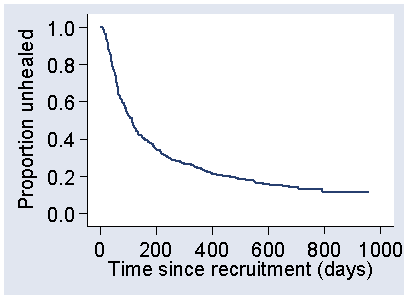d

Although it is called a curve, it is usually shown as a series of abrupt steps, changing sharply at each time when an event takes place. The steps get bigger as we move from left to right, because as we get to longer survival times more observations have been censored and there are fewer at risk. This makes the proportions surviving when there is an event smaller and the steps bigger.

Other than the size of the steps, the curve in Figure 2 does not include any information about the size of the sample used and where people were censored. We often add ticks to indicate the times where there were censored observations, as in Figure 3.

Figure 3. Kaplan Meier survival curve for the SSB group in the VenUS I trial with ticks added to show where censoring took place and the number at risk shown at 100 day intervals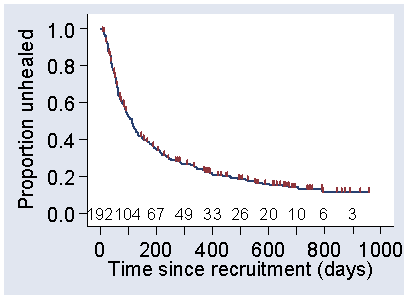d

We can also add the number remaining at risk along the bottom of the graph. In Figure 3, this has been done at 100 day intervals. This goes down not only because of censoring, of course, but because people have healed.

We can add a 95% confidence interval for the survival estimate. This gives what are called Greenwood bounds around the survival curve. Figure 4 shows these for the SSB group.

Figure 4. Kaplan Meier survival curve for the SSB group in the VenUS I trial with Greenwood confidence limits added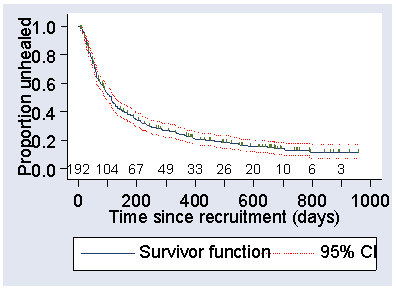d

In Figure 4, the bounds get further apart as we move from left to right. This is because as subjects have been censored, there is less and less information on which to base the estimate. If there is no censoring, they are widest at 50% survival and then get narrower again.

We usually draw these survival curves so that we can compare them and Figure 5 shows the survival curves for the two arms of the VenUS I trial.

Figure 5. Kaplan Meier survival curves for the treatment arms in the VenUS I triald

This shows that the 4LB group appeared to have a lower survival unhealed, i.e. better healing, than did the SSB group. We can also look at the effect of a possible prognostic variable. In VenUS I the area of ulcer at recruitment was expected to be an important predictor of healing. This is a continuous variable, but by grouping the subjects into categories with small, medium, and large ulcers we can see that this is indeed a powerful predictor of healing. As Figure 6 shows, small ulcers healed much more quickly than large ones.

Figure 6. Kaplan Meier survival curves by area of ulcer at baseline in the VenUS I trial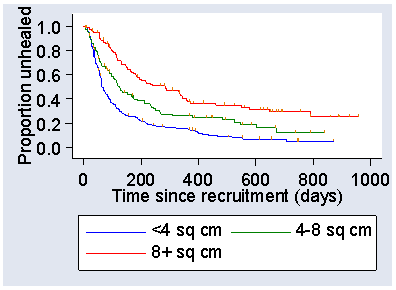d

A graph showing the proportion unhealed may not be what we really want. We can turn the graph upside down and plot the estimated proportion healed instead. This is what was used in the publication of the VenUS I results. Figure 7 shows the plot of the proportion healed against time, by area of ulcer.

Figure 7. Estimated proportion healed (failure function) by area of ulcer in the VenUS I trial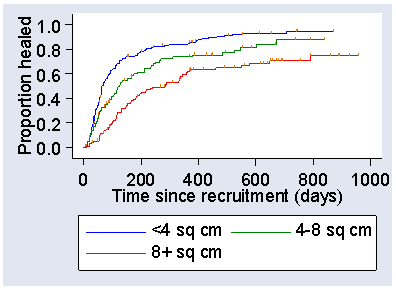d

The proportion healed is, of course, the proportion who do not survive and goes by the unprepossessing name of the failure function, the opposite of the survival function.

## Assumptions of the Kaplan Meier method

The key assumption of the Kaplan Meier method is that the risk of an event is the same for censored subjects as for non-censored subjects. This means:

1. those lost to follow-up during the period of the study are not different from those followed-up to the analysis date,
2. there is no change in risk from start of recruitment to end.

As usual, we also assume that the observations are independent of one another. We can check that risk remains the same by comparing the survival curves for subjects recruited early in the study with those recruited later. Figure 8 shows survival curves obtained by splitting the sample into two parts at the median recruitment number.

Figure 8. Survival curves for the first half and the second half of patient recruitment in the VenUS I trial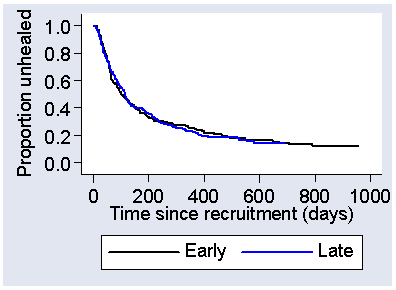d

The curves look almost identical, so there is nothing to suggest that there is a change in risk from start to end of recruitment. It is much more difficult to check those lost to follow-up before the analysis date are not different to those followed to the end. We hope that there are few of these.

The Kaplan Meier survival curve has proved so valuable that Kaplan and Meier (1958) is the mostly highly cited statistical paper to date (Ryan and Woodall, 2005).

## The logrank test

Greenwood standard errors and confidence intervals for the survival probabilities, as shown in Figure 4, are useful for estimates such as five year survival rate. They are not a good method for comparing survival curves. They do not include all the data and the comparison would depend on the time chosen. Eventually, the curves will meet if we follow everyone to the event (Figure 9) and at this point the proportions surviving will be identical.

Figure 9. Two hypothetical survival curves followed until there are no further survivals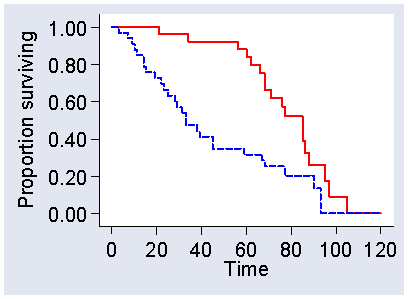d

Instead of the Greenwood method, to compare survival curves we need a method which makes use of the full survival data. There are several significance tests which we can use for this, of which the best known is the logrank test. This is a non-parametric test which makes use of the full survival data without making any assumption about the shape of the survival curve.

The logrank test works like this. The null hypothesis is that at every time the chance of a member of a group experiencing an event is the same for all groups, though the chance of an event may change over time in any way. The alternative hypothesis is that at some time the chance of an event is different in different groups.

We consider only times at which there is an event or a censoring. We put these in ascending order of time of follow-up, as shown in Table 4.

Table 4. Calculation of the logrank test comparing treatment arms in the VenUS I trial
Time SSB 4LD proportion
with events
q = (d1+d2)/(n1+n2)
expected events
in group 1
e1 = n1 × q
n1 c1 d1 n2 c2 d2
0 192 0 0 195 1 0 0/(192+195) 192 × 0/(192+195)
7 192 0 1 194 0 3 4/(192+194) 192 × 4/(192+194)
8 191 1 0 191 0 0 0/(191+191) 191 × 0/(191+191)
10 190 0 1 191 0 0 1/(190+191) 190 × 1/(190+191)
11 189 0 0 191 1 0 0/(189+191) 189 × 0/(189+191)
12 189 0 1 190 0 0 1/(189+190) 189 × 1/(189+190)
13 188 0 1 190 0 1 2/(188+190) 188 × 2/(188+190)
14 187 0 1 189 0 3 4/(187+189) 187 × 4/(187+189)
15 186 0 1 186 0 1 2/(186+186) 186 × 2/(186+186)
17 185 0 0 185 0 1 1/(185+185) 185 × 1/(185+185)
20 185 0 2 184 0 2 4/(187+184) 185 × 4/(187+184)
21 183 0 4 182 1 4 8/(183+182) 183 × 8/(183+182)
. . . . . . . . .
. . . . . . . . .
n1 and n2 = numbers at risk, c1 and c2 = numbers of censored observations, i.e. not followed any longer, d1 and d2 = numbers of events in the two groups

For each time we record for each group the number still at risk, denoted by n1 and n2 in Table 4, the number of events, denoted by d1 and d2, and the number of censorings, denoted by c1 and c2. At each time there is an event or censoring, we find the overall proportion who have an event, which is the total number of events, d1 + d2, divided by the total number at risk, n1 + n2.

We use this to calculate how many events would be expected to happen at that time if there were no difference between the groups in the chance of an event. We multiply the number at risk in the group by the proportion of all subjects at risk who experience the event. For each group, we then subtract the number of events and the number of censored observations at that time, to get the number at risk for the next event. We proceed like this down the table until all the subjects have had an event or been censored. Table 4 shows the start of this calculation only, and shows the calculation of the expected number of events (healings) for the first group, short stretch bandages. We add all these expected numbers of events to give the total number of events expected in the group. For the SSB group the total number of expected events is 160.57. We do the same thing for each group; for the 4LB group the expected total number of events is 143.43.

We actually observed 147 events in the SSB group and 157 events in the 4LB group. The expected numbers of events and the observed numbers have the same sum: 160.57 + 143.43 = 304 = 147 + 157. If the null hypothesis were true, we should expect the observed frequencies to be close to the expected frequencies, just as we would in the chi-squared test for a contingency table (Week 6). We apply the same observed minus expected squared over expected formula for frequencies that we used in the analysis of contingency tables to give a test statistic. For the example, this is equal to 2.46.

If the null hypothesis were true, this would be an observation from a Chi-squared distribution. The degrees of freedom is given by number of groups minus 1 = 2  1 = 1. The probability of getting so high a value of chi-squared with 1 degree of freedom is 0.1, so P = 0.1. The difference in not significant and we do not have evidence that there is any difference in healing between short stretch and four layer bandaging. (This is not the end of the story, however, see below.)

As usual, in practice we do these calculations using a computer program rather than set it all out by hand. There are improvements to the chi-squared formula which may be built in to these programs.

We can have more than two groups in a logrank test. For example, if we group area of ulcer into three categories, <4 sq cm, 48 sq cm, 8 sq cm or more, we get three observed and three expected numbers of healing events:

Area Events observed Events expected
<4 sq cm 176 22.24
48 sq cm 65 70.45
8+ sq cm 63 111.32
Total 304 304.00

Here there are much larger differences between the observed and expected numbers than for treatment arm. If we apply the observed minus expected squared over expected formula to these three pairs, we get chi-squared = 46.84. We have three groups, so the degrees of freedom are 3  1 = 2. The probability of such a large chi-squared value is very small, P < 0.0001, so we have very strong evidence that the area of the ulcer at baseline is a predictor of time to healing.

Like all significance tests, the logrank test requires some assumptions about the data. Like the Kaplan-Meier survival curve, to which it is closely related, we require that observations be independent, that the risk of an event is the same for censored subjects as for non-censored subjects, and that survival is the same for early and late recruitment.

You will also often see it said that the survival curves should not cross. You will find it hard to see why from my description of how the test works. I think that the origin of this idea is that the test is not good at detecting complex differences where risk of an event is higher in one group at beginning and higher in the other group at the end. It is much better at detecting differences where the risk is higher in one group than the other throughout. This assumption is really only relevant to the hazard ratio, described in the next section.

You may be wondering where the logrank test got its name. There are no obvious ranks involved, nor any logs. I confess that I dont understand it either and think of it as just a name.

The logrank test is a nonparametric test and makes no assumptions about the shapes of the underlying survival curves. It is a test of significance only and does not provide any estimate of the size of the difference in survival.

## The hazard ratio

To produce an estimate of the size of the difference in survival, we have to make some assumptions about the shape of the curve. We have to assume that they are similar in some way, so that we can find some numerical value to compare between them. We can use the Greenwood standard errors to find a confidence interval for the difference between the survival probabilities at a given time, but this does not use all the data, events after the chosen time being ignored.

One way to estimate the difference between the survival curves uses the hazard, which is a measure of the chance that a member of the population will have an event at any given time. To be more precise, we find the probability of an event in any small time interval by multiplying the width of the time interval by the hazard at that time. Hazard depends on the survival time, so that it might increase or decrease as follow-up goes on. If we can assume that the survival curves follow the same pattern, then we can assume that if hazard is greater in one group than another at one time, it will also be greater at another. If this is the case, we assume that the hazard in one group is equal to the hazard in the other group multiplied by a constant number, which we will estimate. Thus, if members of one group have twice the risk of an event for members of the other group on the first day, they will also have twice the risk of the event on the second day, twice the risk on the third day, and so on.

The number is called the hazard ratio. It is easy to estimate from the logrank calculations, just using the observed and expected numbers of events. For the 4LB treatment arm compared to the SSB treatment arm, the hazard ratio is

(157/143.43)/(147/160.57) = 1.20.

If the risk of an event is the same in the two groups, the hazard ratio is equal to one, if the risk is lower in the first group the hazard ratio is less than one, if the risk is greater in the first group the hazard ratio is greater than one. Hence we have a small increase in the risk of an event in the 4LB group compared to the SSB group. In this example the event is healing, a good thing, so this shows an advantage to the 4LB group. Of course, this is only an estimate and is subject to error in the estimation, so we need a confidence interval.

Like most ratios, the hazard ratio is much easier to analyse on the logarithmic scale. The approximate standard error for the log hazard ratio is also easy to estimate and for the log 4LB/SSB hazard ratio it is 0.115. The log hazard ratio is 0.177, and the 95% confidence interval is

0.177  1.96 × 0.115 to 0.177 + 1.96 × 0.115 = 0.048 to 0.402.

If we antilog this we get the 95% confidence interval for the hazard ratio, 0.95 to 1.49. This includes 1.00, the null hypothesis value, so it is consistent with the logrank test above.

For the ulcer area, we would need to estimate two hazard ratios, because there are three groups. We choose one of them to be the reference category, as we did in multiple regression equations, with which we compare the other two. Here the obvious choice of reference category is <4 square cm, the group with the smallest ulcers. The hazard ratio for 4-8 sq cm compared to <4 sq cm is 0.64, 95% CI = 0.48 to 0.86 and the hazard ratio for 8+ sq cm compared to <4 sq cm is 0.39, 95% CI = 0.30 to 0.51. Neither of these contain one, reflecting the highly significant relationship shown by the logrank test.

There is more than one way to estimate the hazard ratio, its standard error, and its confidence interval. Different programs may use different approximations and so may not produce exactly the same estimates. Fortunately, the estimates are best for hazard ratios close to one and get less good for very large and very small ratios, but these are going to be so far from one that the inaccuracy is unlikely to affect our interpretation of the data. They are large sample methods and doing this sort of thing for small samples is usually a waste of time as we would not be able to get useful estimates.

## Cox regression

Cox regression is a regression method for survival data, very similar to multiple regression by least squares and logistic regression. As usual, we have an outcome variable, the censored survival times, and some predictor variables with which we hope to predict the survival. Cox regression predicts the hazard ratio for subjects with any given values of the predictor variables compared to subjects for whom the predictor variables are all equal to zero:

log hazard ratio = slope1 × predictor1 + slope2 × predictor2 + ...

There is no intercept in the Cox method, because when all the predictor variables are equal to zero the hazard ratio must be one, and so the log hazard ratio must be zero. We have to assume that the hazard ratio does not depend on the time of follow-up, just as for the simple hazard ratio described above. Cox regression is also known as proportional hazards regression.

For our first example, we shall use area of the ulcer to predict healing in the VenUS I trial. Area of ulcer is a continuous measurement and to estimate a hazard ratio directly we grouped it, throwing away information. If we use Cox regression, we get:

log hazard ratio = 0.0276 × area

The actual calculation of Cox regression equations is quite complicated and is always done using a computer program. As usual in regression, we get a standard error for the coefficient, here = 0.0064, a significance test of the null hypothesis that the slope or coefficient is equal to zero,

z = 0.0276/0.0064 = 4.31, P < 0.001,

and a 95% confidence interval for the slope, 95% CI = 0.0402 to 0.0151. To interpret this more easily, we need to antilog the estimate and its confidence interval. This gives us the hazard ratio = 0.973 per sq cm increase in baseline ulcer area, 95% confidence interval = 0.961 to 0.985 per sq cm increase in baseline ulcer area. It is less than one, so bigger ulcers have lower risk, i.e. less chance of healing. Every increase in area of one cm multiplies the hazard ratio by 0.973.

For another example, we can put treatment arm into our Cox regression, using a variable equal to 1 for 4LB group and 0 for the SSB group. We get hazard ratio = 1.196, z = 1.56, P = 0.119, and the 95% confidence interval = 0.955 to 1.498. We can round this up to 1.120, P = 0.1, 95% CI = 0.96 to 1.50. In this analysis SSB is the reference treatment, so the risk of healing in the 4LB arm is estimated to be between 0.96 and 1.50 times that in the SSB arm.

If we compare the results of the Cox regression for treatment to the hazard ratio found in the previous section, apart from a few rounding errors in the calculation, we get the same answer. The test of significance is also very similar to the logrank test, which gave us chi-squared = 2.46 with one degree of freedom. This has P = 0.117, compared to P = 0.119 for the Cox regression. This is not due to rounding errors, as the logrank test does not give quite the same P value as Cox regression. The difference is tiny and of no practical importance. As always, when there is more than one way to do something we should choose one and stick to it, not try them all to see which result we like best.

The great strength of Cox regression compared to log rank tests and simple hazard ratios is that we can have more than one predictor variable. For the VenUS I trial, we can improve the estimate of the treatment effect by including prognostic variables in the regression, just as we did for the asthma nurse trial in Week 7. Area of the ulcer is an obvious variable to use, as it is such a good predictor of the outcome. The Cox regression gives:

log hazard ratio = 0.286 × area + 0.238 × group
95% CI          0.041 to 0.015     0.013 to 0.464
P < 0.001             P=0.038

If we antilog these, the hazard ratio for an increase in ulcer area of one square cm = 0.972 (95% CI 0.960 to 0.985) and for treatment group is 1.27 (95% CI 1.01 to 1.59, P=0.04). Compare this to the estimated hazard ratio when we ignored the area, 1.20, (95% CI = 0.96 to 1.50, P = 0.1). Using the area produces a much better estimate of the treatment effect, because it explains a lot of the variation in survival.

In the paper reporting the results of the VenUS I trial the treatment difference was adjusted for ulcer area, duration of the ulcer, previous episodes of venous ulcers, weight, ankle mobility, and trial centre (Nelson et al., 2004). The authors reported that:

... when prognostic factors were included in a Cox proportional hazards regression model, ulcers treated with the short-stretch bandage had a lower probability of healing than those treated with the four-layer bandage: hazard ratio 0·72 (95 per cent confidence interval 0·57 to 0·91). (Nelson et al., 2004).

This uses the four-layer bandage as the reference group, and the hazard ratio is the reciprocal of ours. To make ours comparable, we take reciprocals: 1/1.27 = 0.79, 95% CI 1/1.59 = 0.63 to 0.99). Including more variables made the treatment effect estimate a little bigger.

You may notice that the estimate of the hazard ratio is increased by the adjustment, from 1.20 to 1.27 after adjusting for baseline area and to 1/0.72 = 1.39 after adjusting for the other prognostic variables also. The width of the confidence intervals changes very little, the ratios of upper limit to lower limit being 1.57 unadjusted, 1.57 after adjusting for area, and 1.60 after adjusting for all prognostic variables. This is typical of Cox models. Logistic regression behaves in the same way. The reasons for this are described by Ford and Norrie (2002).

There are several different regression methods for survival data. Most of them require us to assume that the survival curve has a particular shape. This is not going to be a particularly severe problem when we are dealing with nice, simple, uniform objects like light bulbs (we can use survival analysis to decide when to replace them) but does not work well with complicated things like sick people. The great breakthrough of Cox regression was that we do not need to assume any particular shape for the survival curve. This is why it is the one almost always used in health research. Cox regression is described as semi-parametric: it is non-parametric for the shape of the survival curve, which requires no model, and parametric for the predicting variables, fitting an ordinary linear model. According to Ryan and Woodall (2005), Cox (1972) is the second mostly highly cited statistical paper to date.

There are several assumptions we must make about the data for Cox regression:

1. observations are independent,
2. as for Kaplan Meier, the risk of an event is the same for censored subjects as for non-censored subjects,
3. the proportional hazards model applies,
4. there are sufficient data for the maximum likelihood fitting and large sample z tests and confidence intervals.

For the last assumption, a rule of thumb is that there should be at least 10 events per variable in the model, preferably 20. Things might get very unreliable if we tried to fit Cox regression models with smaller samples. For the VenUS I data, there were 304 events, and we fitted two variables, giving 152 events per variable, which is ample. Nelson et al. (2004) used treatment, ulcer area, duration of the ulcer, previous episodes of venous ulcers, weight, ankle mobility, and trial centre. There were nine centres, which would require eight dummy or indicator variables to represent them. There were 14 variables altogether, 304/14 = 21.7 events per variable, so this analysis did indeed satisfy the more stringent rule of thumb.

There are several ways to check the proportional hazards assumption. We can look at the Kaplan Meier plots to see whether they look OK, e.g. that they do not cross. It is not very easy to see other than gross departures. If we look at the survival curves for treatment (Figure 5) they appear to be almost identical for the first 50 days of follow-up, then to diverge, and to come together against about 650 days. Whether this means anything is difficult to say, but the hazard ratio may not be uniform. For the three area groups (Figure 6), the curves for <4 and 4-8 sq cm appear identical for the first 50 days then diverge, otherwise things look OK.

## References

Clements MS, Mitchell EA, Wright SP, Esmail A, Jones DR, Ford RPK. (1997) Influences on breastfeeding in southeast England. Acta Paediatrica 86, 51-56.

Cox DR. (1972) Regression Models and Life Tables. Journal of the Royal Statistical Society, Series B 34, 187-220.

Ford I and Norrie J. (2002) The role of covariates in estimating treatment effects and risk in long-term clinical trials. Statistics in Medicine 21, 28992908.

Kaplan EL and Meier P. (1958) Nonparametric Estimation from incomplete observations. Journal of the American Statistical Association 53, 457-81.

Luthra P, Bland JM, Stanton SL. (1982) Incidence of pregnancy after laparoscopy and hydrotubation. British Medical Journal 284, 1013.

Mitchell EA, Bland JM, Thompson JMD. (1994) Risk factors for readmission to hospital for asthma. Thorax 49, 33-36.

Nelson EA, Iglesias CP, Cullum N, Torgerson DJ. (2004) Randomized clinical trial of four-layer and short-stretch compression bandages for venous leg ulcers (VenUS I). British Journal of Surgery 91, 1292-1299.

Petroni ML, Jazrawi RP, Pazzi P, Zuin M, Lanzini A, Fracchia M, Facchinetti D, Alvisi V, Ferraris R, Bland JM, Heaton KW, Podda M, Northfield TC. (2000) Risk factors for the development of gallstone recurrence following medical dissolution. European Journal of Gastroenterology & Hepatology 12, 695-700.

Ryan TP and Woodall WH. (2005) The most-cited statistical papers. Journal of Applied Statistics 32, 461-474.